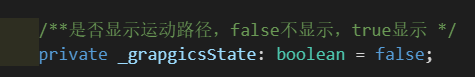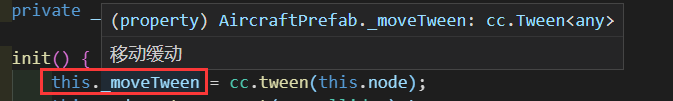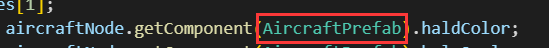# [muzzik分享]：浅谈自己的编程风格｜社区征文

## # 命名结构

• boolean：
``````let open_b: boolean;
let touch_b: boolean;
``````
• string：
``````let name_s: string;
let time_s: string;
``````
• number：
``````let count_n: number;
let time_n: number;
``````
• object：若是类型名超过 4 个字符则直接省略或者简写
``````let temp_node: cc.Node;
let temp_anim: cc.Animation;
``````
• any 或不清楚类型：那就直接省略
``````let temp: any;
``````
• array：一维便是一 s，对象类型用 a 代替
``````// 基础数据类型数据
let temp_ss: string[];
let temp_ns: number[];
let temp_bs: boolean[];

// 对象数组
let node_as: cc.Node[];

// 多维数组
let node_ass: cc.Node[][];
let node_asss: cc.Node[][][];
``````

## # 命名规则

### - Class

• private 成员/函数：下划线开头，代表内部访问成员，从学习C++遗留至今
``````private _test: any;
``````
• protected 成员/函数：下划线开头，代表内部访问成员，如上
``````protected _test: any;
``````
• public 成员/函数：不用下划线开头，代表内外部访问成员
``````public test: any;
``````

### - Function

• 参数：下划线结尾，代表外部参数，能一眼分辨函数外部参数和函数内部变量的区别
``````class test {
public func(test_b_: boolean, args_as_: any[]): void { ... }
}
``````

### - 复数变量

• 直接在第二个及之后变量末尾追加数字，为什么是第二个？因为有些时候你不确定是否会出现复数变量
``````let temp_n: number;
let temp2_n: number;

// 循环示例，用的最多!!!，也是最方便的
this.node.children.forEach((v, k_n)=> {
v.children.forEach((v2, k2_n)=> {
});
});
``````

## # 代码块划分

``````function temp() {
// 这里是代码块1
{
let temp_n = 0;
// 这里是代码块2
{
let temp_n = 0;
}
}
}
``````

## # 文件命名

``````// assets/script/main/login

``````

## # 模块结构

``````// 存放模块内部使用的接口，类型，枚举，常量等
module _test { ... }

class test { ... }

// 存放模块内部和外部使用的接口，类型，枚举，常量等
export module test_ { ... }

export default test;
``````

## # 模块集成

``````// ui_a.ts
export default a;

// ui_b.ts
export default b;

// ui_export.ts
export { a, a_ } from "./a";
export { b, b_ } from "./b";

// ui.ts
import * as ui from "./ui_export";
export default ui;
``````
2赞

1. 命名结构：变量在前，前小写后大写，类型在后，类型必须写，不清楚的any代替。
1.1.const goHome: string = ‘’;
1.2.let goGame: boolean = true;
1.3.let goGame: any = 11111;
2. Class:方法定义
2.1.private _test: number = 1
2.2. protected test: string = ‘’;
2.3. public test: string[] = [];
3. 预制体命名：大写开头，Prefab后缀结尾
3.1. TipViewPrefab
4. TS文件命名：大写开头
4.1. 关联预制体时，和预制体一样的名字，TipViewPrefab.ts
4.2. 管理器：TipManager.ts
5. 图片资源命名：ui开头，数字结尾
5.1. ui1…ui*，分目录归类管理
6. 补充下类方法
6.1.方法需要传参必须指定类型：private _test(sprite: cc.Sprite, node: cc.Node) {}
6.2.方法非参必须定义不能带？号：private _test(num: number, color: cc.Color = cc.Color.RED)
6.3. 方法自定义类型，必须先定义接口：interface TEST {num: string} private _test(num: TEST) {}
7.1. 方法注释：7.2. 属性注释：7.3. 行内注释：

7.4. 正确使用注释，鼠标移动上去都会显示7.8. getComponent()访问挂载脚本时，建议使用引用的方式，可以显示对应的注释，但一定要注意循环引用：